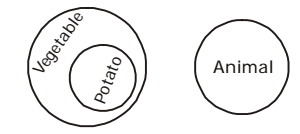## Logical Venn Diagram

#### Logical Venn Diagram

1. In the above figure, the circle stands for employed, the square stands for social worker, the triangle stands for illiterate and the rectangle stands for truthful. Employed, truthful and illiterate social workers are indicated by which region?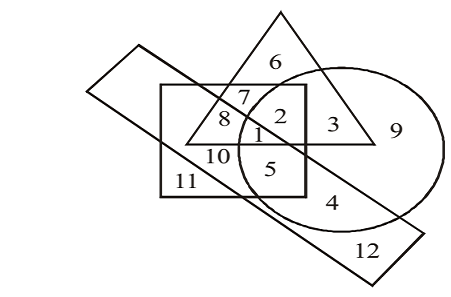1. From above given diagram , we can see that
Employed, truthful and illiterate social workers would be indicated by the region common to all the four geometrical figures. Such region is marked '1’.

##### Correct Option: D

From above given diagram , we can see that
Employed, truthful and illiterate social workers would be indicated by the region common to all the four geometrical figures. Such region is marked '1’.
Hence , required answer is 1 .

1. In the given diagram, circle represents professionals, square represents dancers, triangle represents musicians and rectangle represents Europeans. Different regions in the diagram are numbered 1 to 11. Who among the following is neither a dancer nor a musician but is professional and not a European?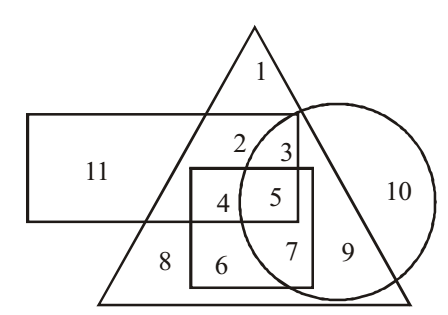1. As per the given above diagram , we get
The required region should be present only in circle. Such region is marked ‘10’.

##### Correct Option: C

As per the given above diagram , we get
The required region should be present only in circle. Such region is marked ‘10’.
From above it is clear that option ( C ) is required answer.

1. Which one of the following diagrams represents the correct relationship among day, week, year?

1. We can say that
Both Day and week belong to year . Year represents the biggest circle ,Day represents the smallest circle , and week represents the middle circle .As shown in figure .

##### Correct Option: A

As we know that
Both Day and week belong to year .Year represents the biggest circle ,Day represents the smallest circle , and week represents the middle circle .As shown in figure .
From above it is clear that required answer is option ( A ) .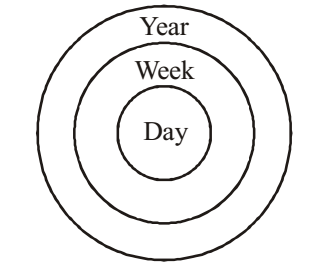1. Which of the following diagram represents men, women and pregnant women?

1. According to above given question , we know that
Men are different from women.

##### Correct Option: C

According to above given question , we know that
Men are different from women.
Hence , option ( C ) gives the best relationship between men, women and pregnant women .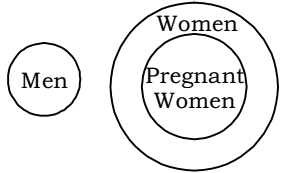1. Which figure represents the relation among animals, vegetables and potatoes?

1. According to above given question , we can say that
Potato comes under the class vegetable.
But animal is different from them.

##### Correct Option: C

According to above given question , we can say that
Potato comes under the class vegetable.
But animal is different from them.
It is clear that option ( A ) represents the best relationship between animals, vegetables and potatoes . As shown in given venn - diagram .